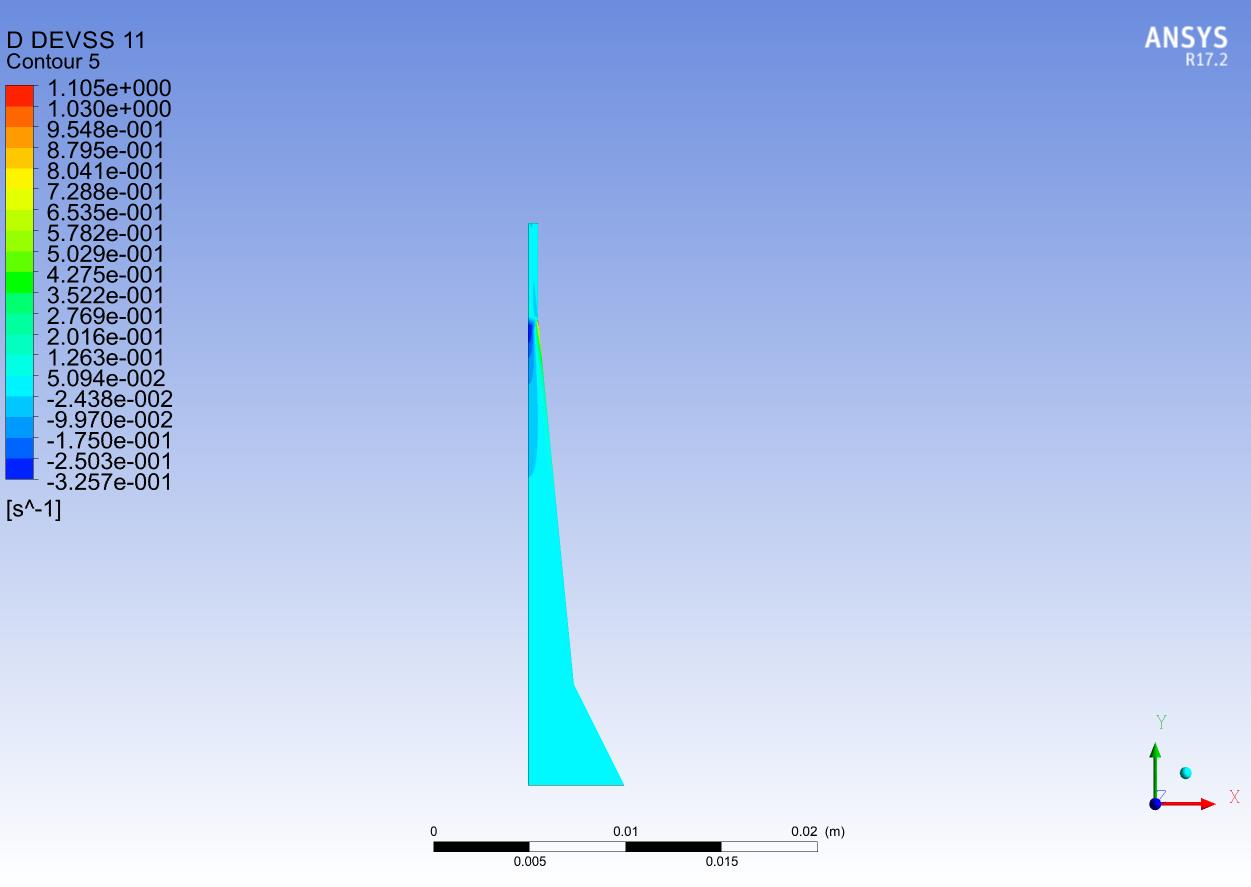# The field management for 2D differential viscoelastic simulation!

I  am using polyflow to perform a 2D differential viscoelastic simulation of the extrusion die, using the DEVSS and SU methods. In field management, I added the field D DEVSS. Then i obtain the DEVSS results in the cfd-post. But i have a question that what the physical meaning of DEVSS is?

Regards!

••• edited April 2020

When the EVSS technique is used for solving the viscoelastic constitutive equation, the extra-stress tensor T is written as T = S + 2.η.D, while the constitutive equation is rewritten accordingly. Hence there are two tensors, S and D. Since they are used within the context of the EVSS technique, they have been named S EVSS and D EVSS.
[See also: D. Rajagopalan, R.C. Armstrong and R.A. Brown, Finite element methods for calculation of steady viscoelastic flows using constitutive equations with a Newtonian viscosity, J. non-Newt. Fluid Mech., 36 (1990) 159-192]

When the DEVSS technique is used for solving the viscoelastic constitutive equation, the constitutive equation for the extra-stress tensor T is kept unchanged, it has been named T DEVSS. Only the momentum equation is modified, a viscous term expressed in terms of velocity gradient unknowns is added, while a counterpart expressed in terms of rate-of-deformation tensor unknowns is removed.
A new tensor is thus calculated, the rate-of-deformation tensor. Within the context of the DEVSS technique, it has been named D DEVSS.
[See also: R. Guénette and M. Fortin, A new mixed finite element method for computing viscoelastic flow, J. non-Newt. Fluid Mech., 60 (1995) 27-52]

• Thanks for your kind reply. And the DEVSS is divided into 4 parts: DEVSS11, DEVSS12, DEVSS22, DEVSS33. So the DEVSS is the sum of these components?

• edited April 2020

Those are the component of the tensor.   Lets say a tensor T

What is the meaning of the component Tij of a stress tensor?

It is the j-th component of the force applied on an infinitesimal element, whose orientation is along the i-direction. Note that individual components of a tensor are not really relevant, on the same way as individual components of a velocity vector are not really more relevant either...

What is the meaning of T11, T12, T22, T33 in a 2D axisymmetric flow?

The reference frame is (r,z), both directions being referred to as 1 and 2. They are respectively the radial and axial directions. A third direction (theta or t), referred to as 3, is also considered : the hoop, azimuthal or circumferential direction. Hence, T11 refers to Trr, T22 refers to Tzz, T12 refers to Trz, and T33 refers to as Ttt.